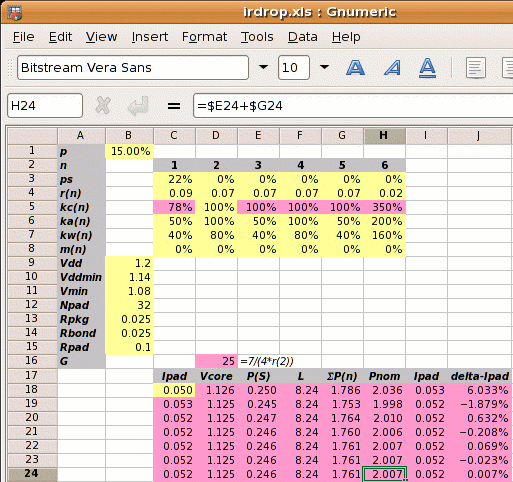An alternative to calculating the supply strap width given the core power consumption is to calculate the core power given the width of the supply straps. We will use a power strap allocation percentage p=15% with the power strap ratios and metal resistivities of the previous example to find the maximum core power.

Step 1: Estimate Vcore using Ipad=50mA:

Vcore =
1.14×(1−2×0.050×(0.025+0.025+0.1)⁄1.2
1.126V

Step 2: Calculate the reference power supply conductance G:

G =
 7 4×r2

7 ⁄ (4 × 0.07) =  25 mhos

Step 3 is to set out the values of kan, kwn, kcn and mn for each metal layer, and use these to calculate the value of L.

metal layer 1 2 3 4 5 6
kan  50% 100%  50% 100%  50% 200%
power metal allocated coefficient
kwn  80%  80%  80%  80%  80%  80%
power metal used coefficient
kcn  78%¹ 100% 100% 100% 100% 350%²
conductivity coefficient
mn   0%   0%   0%   0%   0%   0%
core area blocked
¹78%=.07/.09; ²350%=.07/.02

From which

 L = kw1kc1(1-ps)(1-m1(1-ka2p)(1-ka3p))+ kw2kc2(1-m2(1-ka2p)(1-ka3p))+ kw3kc3(1-m3(1-ka2p)(1-ka3p))+ kw4kc4(1-m4(1-ka2p)(1-ka3p))+ kw5kc5(1-m5(1-ka2p)(1-ka3p))+ kw6kc6(1-m6(1-ka2p)(1-ka3p)) = ( 0.24 + 0.8 + 0.4 + 0.8 + 0.4 + 5.6 ) = 8.24

Step 4: Calculate Pnom. Pnom depends on the value of Ipad which we don't know, so we start with Ipad=50mA which gives us Vcore, then Pnom and a new value of Ipad. We use a spreadsheet to iterate to the solution. The yellow squares mark user input and the pink squares are calculated values. The first estimate for Pnom is shown below.

Pnom =
 { (Vcore−Vmin)×Vdd2 } ×G×(kc1×ps+p×L) Vddmin
 { (1.126−1.08)×1.22 } ×25×(0.78×0.22+0.12×8.24) 1.14
1.445×(0.173+1.236) = 2.036W

The iteration leads to the solution of Pnom=2.007W. This is slightly higher than the 2W of the previous example, which we expect because the power strap allocation percentage is slightly higher at 15% instead of 14.92%.

Design Attribute Typical Value
p metal power supply allocation percentage 15%¹
ps the fraction of metal-1 in the standard cells dedicated to power supplies 22% (for vsclib)
r1 resistivity of metal layer 1 in ohms per square 0.09Ω per sq.
r2-5 resistivity of metal layers 2-5 in ohms per square 0.07Ω per sq.
r6 resistivity of metal layer 6 in ohms per square 0.02Ω per sq.
ka1,3,5
 user defined   ratio of metal layers 1,3,5 allocated to power metal-2 allocated to power
50%
ka4
 user defined   ratio of metal layer 4 allocated to power metal-2 allocated to power
100%
ka6
 user defined   ratio of metal layer 6 allocated to power metal-2 allocated to power
200%
mn percentage of metal layer n blocked to power straps 0%
Vdd the nominal supply voltage 1.2V
Vddmin the minimum supply voltage, 5% less than nominal 1.14V
Vmin the desired voltage at the centre of the die, 10% less than the nominal 1.08V